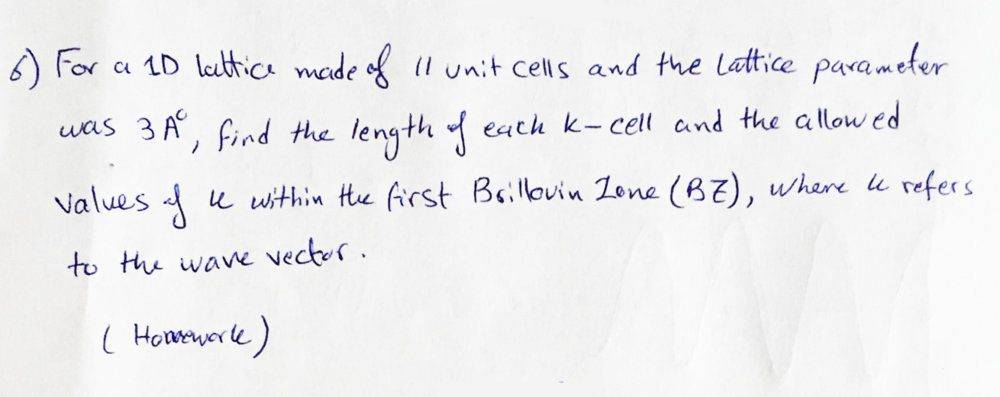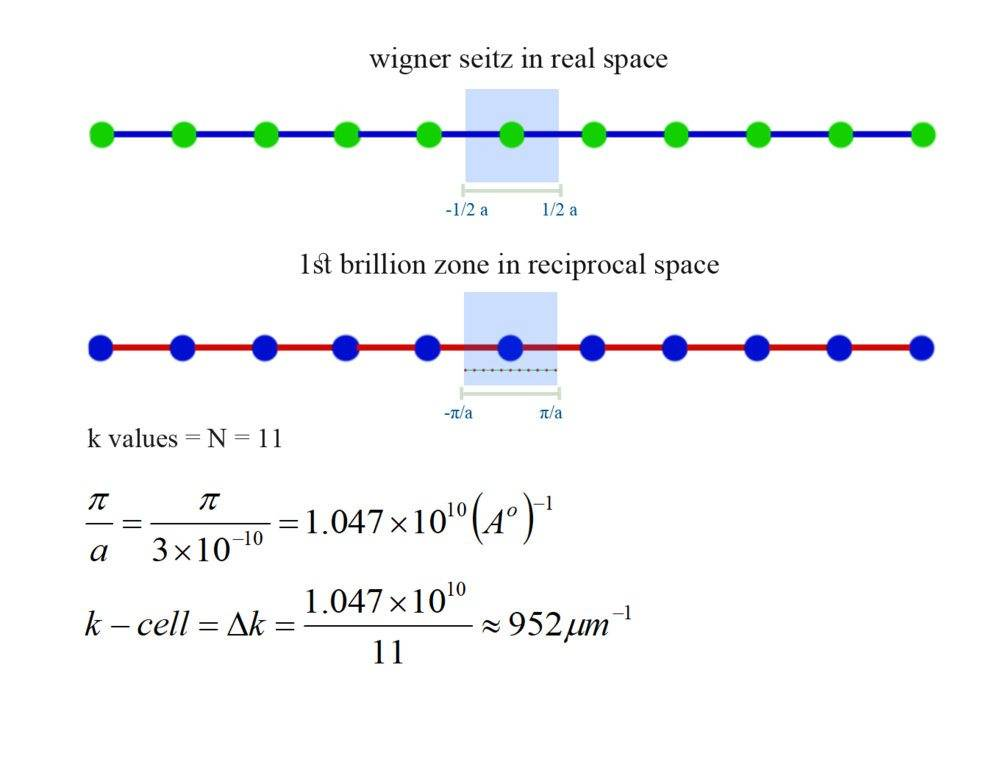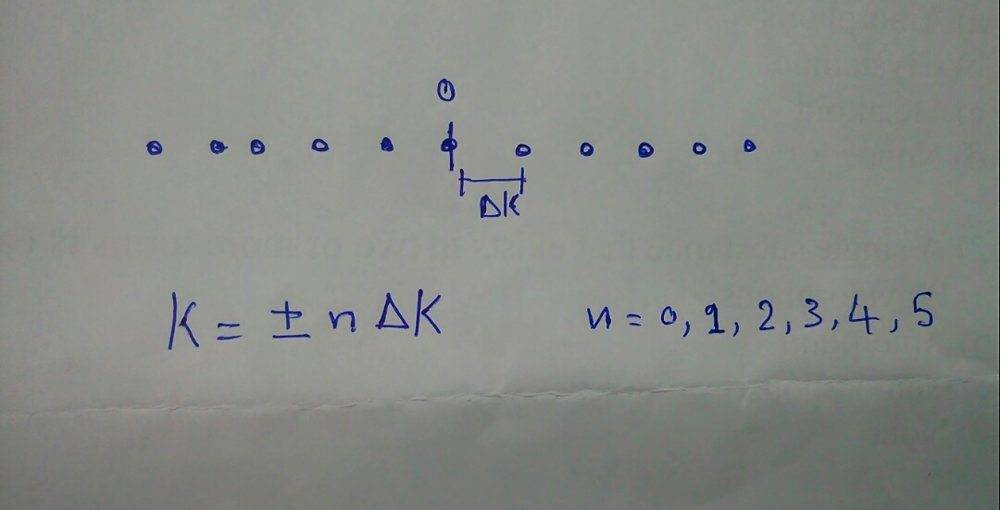# Number of k-cells for a 1D lattice in reciprocal space

Homework Statement:
find the numer of k-cells for a 1d lattice in reciprocal space ?
Relevant Equations:
attached
hi guys
our solid state professor sent us a work sheet that included this example :i solved it not sure its correct tho :is it that simple , or this is not the right approach for it ?

## Answers and Replies

ehild
Homework Helper
Homework Statement:: find the numer of k-cells for a 1d lattice in reciprocal space ?
Relevant Equations:: attached

hi guys
our solid state professor sent us a work sheet that included this example :
View attachment 260534
i solved it not sure its correct tho :
View attachment 260537
is it that simple , or this is not the right approach for it ?
It is the right approach, but the result is not correct.
What are the allowed values of k? What is the length of each cell?

It is the right approach, but the result is not correct.
What are the allowed values of k? What is the length of each cell?
i did something wrong , i guess the length of the brillion zone is
$$\frac{2π}{a} = \frac{2π}{3*10^-10} = 2.094*E10 A^-$$
$$dK =\frac{2.094E10}{11} =1.9*10^9 A^-$$
the allowed values of k i guess would be multiples of dk ,1dk,2dk ....

Last edited:
ehild
Homework Helper
i did something wrong , i guess the length of the brillion zone is
$$\frac{2π}{a} = \frac{2π}{3*10^-10} = 2.094*E10 A^-$$
$$dK =\frac{2.094E10}{11} =1.9*10^9 A^-$$
the allowed values of k i guess would be multiples of dk ,1dk,2dk ....
It is better for the length, but not the allowed values. Is k=0 allowed? And negative k values? And what about the magnitude? Is 1/A° really 10^9 A°^-?

It is better for the length, but not the allowed values. Is k=0 allowed? And negative k values? And what about the magnitude? Is 1/A° really 10^9 A°^-?
what is the way for knowing which k is allowed ? a little hint
2π/3*10^-10 = 2.094*10^10 and since "a" has units of A so this value has units of is A^-1
so k = 2.094*10^10/11 = 1.9*10^9 still has units of A^-1 ?
what am i doing wrong

ehild
Homework Helper
what is the way for knowing which k is allowed ? a little hint
2π/3*10^-10 = 2.094*10^10 and since "a" has units of A so this value has units of is A^-1
so k = 2.094*10^10/11 = 1.9*10^9 still has units of A^-1 ?
what am i doing wrong
You converted Angstroms to meters. 1 A° =10-10 m. What is 2π/(3A°) then? Always write out the units!

You converted Angstroms to meters. 1 A° =10-10 m. What is 2π/(3A°) then? Always write out the units!
Sorry My bad :), what does he mean by allowed value of k is it just multiples of dk like I just wrote or something else
How would I know which values is allowed say 0 or negative like you mentioned

k could have values of = 2nπ/a but if i let that be k would exceed the length of the first brillon zone ?

ehild
Homework Helper
k could have values of = 2nπ/a but if i let that be k would exceed the length of the first brillon zone ?
No, the allowed k values depend on the size of the lattice. You remembered that in your first post.
There are as many allowed k values in the first Brillouin Zone, as Brawais cells in the real crystal, that is 11 in this problem. You know the size of the cells in the reciprocal lattice (what did you get at last?) : the Brillouin Zone is the Wigner-Seitz cell in that space. If the lattice points in the real crystal are at x= 0, +-a, +-2a... those of the reciprocal lattice are at n2pi/a. The Wigner Seitz cell in he real lattice is symmetric to x=0, the edges of the first one are at -a/2 and a/2. The First Brillouin Zone in the reciprocal lattice is analogous to that.
k means a point in the momentum space (reciprocal space) as x means a point in the real space. k is the wave vector of a wave travelling in the crystal. Two waves are identical if their phase differ by a reciprocal cell vector. You certainly learnt about it!

No, the allowed k values depend on the size of the lattice. You remembered that in your first post.
There are as many allowed k values in the first Brillouin Zone, as Brawais cells in the real crystal, that is 11 in this problem. You know the size of the cells in the reciprocal lattice (what did you get at last?) : the Brillouin Zone is the Wigner-Seitz cell in that space. If the lattice points in the real crystal are at x= 0, +-a, +-2a... those of the reciprocal lattice are at n2pi/a. The Wigner Seitz cell in he real lattice is symmetric to x=0, the edges of the first one are at -a/2 and a/2. The First Brillouin Zone in the reciprocal lattice is analogous to that.
k means a point in the momentum space (reciprocal space) as x means a point in the real space. k is the wave vector of a wave travelling in the crystal. Two waves are identical if their phase differ by a reciprocal cell vector. You certainly learnt about it!
thanks for helping , so there are only 11 allowed values of k with an interval between them = 2π/Na = 0.19A.

ehild
Homework Helper
thanks for helping , so there are only 11 allowed values of k with an interval between them = 2π/Na = 0.19A.
Yes, there are 11 allowed values of k in the first Brillouin zone (what is its size?) The problem asks you to give these k values.

i would say k = 0.19 , 0.38 ,0.57 ,0.76 ,.... up to 2.09 as an integer multiples of dk

ehild
Homework Helper
i would say k = 0.19 , 0.38 ,0.57 ,0.76 ,.... up to 2.09 as an integer multiples of dk
The Brillouin zone is a Wigner-Seitz cell, symmetric to k=0. Is k=0 also allowed? And what is the unit of k? It is not A as you wrote.

The Brillouin zone is a Wigner-Seitz cell, symmetric to k=0. Is k=0 also allowed? And what is the unit of k? It is not A as you wrote.A^-1

ehild
Homework Helper
Nice!Do not forget, that k is a wave-vector, and the wave can travel forward and backward, so k can be both + and -

•patric44 and jim mcnamara
Thank you so much

ehild
Homework Helper
Thank you so much
you are welcome.